Surface Area of Common Solids
 Home > Lessons > Surface Area Search | Updated December 18th, 2018
Introduction

In this section, you will learn how to calculate the surface area of common solids to include:

Within our area section, we had to provide a definition for the meaning of area. That definition rested upon the square -- particularly a unit square. A unit Square can be 1" x 1" or 1 yd x 1 yd or 1 ft x 1 ft or a square by some other unit.Unit Square

We saw the area of a figure was nothing more than the sum of all unit squares of a figure. For the surface area of a solid, there is a similar definition, but it applies to the exterior surfaces of the solid. The definition of surface area is the sum of all unit squares that fit on the exterior of a solid.

SA = 2(lw + hl + hw)General Prism

This is the best figure to begin with when investigating surface area. It is the most simple figure of all the solids. It is also a figure most people have personal experience due to either wrapping or opening gifts.

All the surfaces of a prism are rectangular. This makes calculating the areas of these surfaces very easy to do. The area of rectangles have been discussed in another section, which is available for review before proceeding, if necessary.

As the diagram below indicates, there are six surfaces to a rectangular prism. There is a front, back, top, bottom, left, and right to every rectangular prism. The surface are of a prism is nothing more than the sum of all the areas of these rectangles.Using the labeling of the general prism diagram above, a formula can be created for dealing with the surface area of prisms. Let's calculate the area of each surface.

 Prism Surface Area Formula Top : lw Bottom : lw Front : hl Back : hl Left : hw Right : hw Total : lw + lw + hl + hl + hw + hw : 2lw + 2hl + 2hw : 2(lw + hl + hw)

Example 1: Given l = 4 yds, w = 2 yds, and h = 5 yds, the surface area would be...

SA = 2(lw + hl + hw)
SA = 2[(4 yds)(2 yds) + (5 yds)(4 yds) + (5 yds)(2 yds)]
SA = 2(8 yds2 + 20 yds2 + 10 yds2)
SA = 2(38 yds2)
SA = 76 yds2

Example 2: Given l = 6 mm, w = 9 mm, and h = 8 mm, the surface area would be...

SA = 2(lw + hl + hw)
SA = 2[(6 mm)(9 mm) + (8 mm)(6 mm) + (8 mm)(9 mm)]
SA = 2(54 mm2 + 48 mm2 + 72 mm2)
SA = 2(174 mm2) = 348 mm2ideo: Surface Area of a Rectangular Prismuizmaster: Surface Area of Prisms

SA = 2πr2 + 2πrh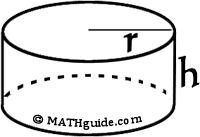General Cylinder

A cylinder has a total of three surfaces: a top, bottom, and middle. The top and bottom, which are circles, are easy to visualize.

The area of a circle is πr2. So, the area of two circles would be πr2 + πr2 = 2πr2.

The third surface, the lateral surface area, is less easy to visualize for the purposes of calculating its area, especially since it does not appear to be in a shape that fits a known area like a triangle or parallelogram. The surface being referred to is the curved wall of the cylinder.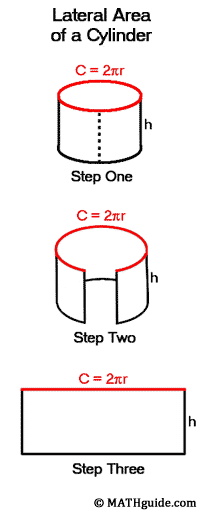We can manipulate the curved wall of a cylinder to produce a recognizable shape. Imagine starting with a cylinder, like a typical can, and then cutting the can up its wall. From the bottom to the top of the can, a cut is made. See the dashed segment in step one of the diagram to the right.

Now, from the newly cut edge, the cylinder wall will be spread open. Almost like opening double doors to a fancy home, the walls spread apart. See step two of the same diagram.

When the cylinder's wall is completely open, it takes the form a very recognizable, basic shape. It is a rectangle. Fortunately, the area of a rectangle is easy to calculate. But we need to go back to the original cylinder for a moment before we calculate the rectangle's area.      The top of the original cylinder is a circle. We know that the distance around a circle is called its circumference, C = 2πr. The circumference has been marked in red.

When the cylinder wall is completely open, we see that the circumference of the circle becomes the length of the final rectangle. The dimensions of the rectangle are the circumference, C = 2πr, and the height of the cylinder, h. So, the area of the rectangle is A = l x w = C x h = 2πr x h = 2πrh.

Finally, if we put all the three surfaces together, we can arrive at the formula for the complete surface area of a cylinder. The top, the bottom, and the walls of the cylinder (the lateral surface area) determine the total surface area. Those values are πr2 + πr2 + 2πrh = 2πr2 + 2πrh. Sometimes this expression is written as 2πr(r + h) in certain geometry texts. [Can you see how 2πr2 + 2πrh is equal to 2πr(r + h) by the distributive property?]

Example 1: If r = 6 ft and h = 4 ft, then the surface area would be...

SA = 2πr2 + 2πrh
SA = 2(3.14159)(6 ft)2 + 2(3.14159)(6 ft)(4 ft)
SA = 2(3.14159)(36 ft2) + 2(3.14159)(24 ft2)
SA = 226.19448 ft2 + 150.79632 ft2
SA = 376.9908 ft2
SA = 377.0 ft2 (rounding to the nearest tenth)

Example 2: If r = 5 cm and h = 2 cm, then the surface area would be...

SA = 2πr2 + 2πrh
SA = 2(3.14159)(5 cm)2 + 2(3.14159)(5 cm)(2 cm)
SA = 2(3.14159)(25 cm2) + 2(3.14159)(10 cm2)
SA = 157.0795 cm2 + 62.8318 cm2
SA = 219.9113 cm2
SA = 219.9 cm2 (rounding to the nearest tenth)ideo: Surface Area: Cylinderuizmaster: Surface Area of Cylinders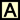ctivity: Cylinder Surface Area

SA = s2 + 2sl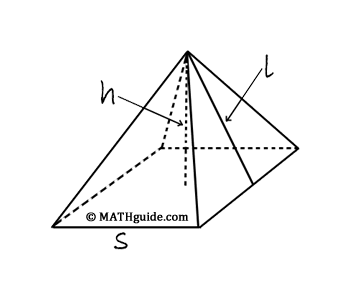Pyramids that have a square base have a total of five surfaces. To determine the shapes of those surfaces, we will start with a pyramid from step one below. If we cut along the lateral edges of the pyramid, we can allow the figure to flatten out in step two below. From step two, the individual figures are easily identified as a square and four triangles.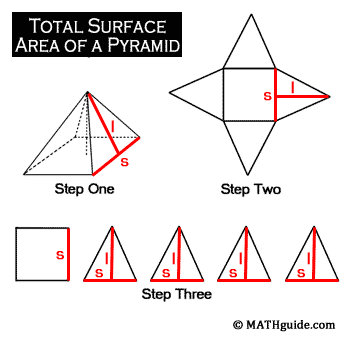We can use the area formulas for a rectangle and a triangle to determine the complete formula for the surface area of the pyramid. The square (or base of the solid) has an area that can be calculated by multiplying its length times its width. Since those dimensions are equal, the area is s x s = s2.

Now we need to calculate the area of the remaining surfaces. The remaining surfaces happen to form the lateral surface area of the pyramid, which are triangles. The area formula for a triangle is its base times its height divided by two. In the case of one of the triangles above it would be s x l ÷ 2. However, there are four triangles. This would make the total lateral surface area equal to four times the area of one triangle, or 4 x s x l ÷ 2. Upon simplifying the expression, we get 2sl.

The total surface area of the pyramid is equal to the area of the base plus its lateral surface area or s2 + 2sl. So, given the base length of the pyramid, s, and its slant height, l, the total surface area is not a difficult computation. Yet, when dimensions are provided for a pyramid, one is rarely provided with the slant height, l. Instead, the height of the pyramid is given. This is an important difference, as one is always larger than the other.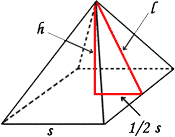Not knowing the slant height makes calculating its value the first goal for finding the lateral area of a pyramid. The diagram to the right will provide insight into finding a relationship for calculating the slant height.

A right triangle, which rests internally within the pyramid, has been highlighted. The hypotenuse (longest side) is the slant height of the pyramid, l. This is the length we need to know in order to calculate the surface area of the pyramid. The height of the pyramid is a leg of the right triangle. The base of the right triangle is half the length of the base edge of the pyramid, s. At this point, we would use The Pythagorean Theorem to calculate the slant height.

Pythagoras said leg2 + leg2 = hypotenuse2. In the case of our right triangle, we would get (1/2 s)2 + h2 = l2. Solving for the slant height would then depend on our ability to use the Pythagorean Theorem.

To review the process, there is an outline of the steps that need to be taken:

1. Determine the height, h, and base edge, s, of a given pyramid. Make sure their values are in the same unit measure (feet, centimeters, ...).
2. Use The Pythagorean Theorem to calculate the slant height, l, of the pyramid.
3. Calculate the area of the base, s2.
4. Calculate the lateral surface area, 2sl.
5. Calculate the total surface area by adding the base area to the lateral area, s2 + 2sl.
6. Report the final surface area value using square units.

Here are two examples that demonstrate how to calculate the total surface area of a pyramid.

Example 1: Given: s = 8 m and h = 3 m.

First we calculate the slant height using The Pythagorean Theorem and half the base length.
32 + 42 = l2
9 + 16 = l2
25 = l2
l = 5 m

The surface area would then require use of the formula...
SA = s2 + 2sl
SA = (8 m)2 + 2(8 m)(5 m)
SA = 64 m2 + 80 m2
SA = 144 m2

Example 2: Given: s = 7 in and h = 6 in.

First we calculate the slant height using The Pythagorean Theorem and half the base length.
(3.5)2 + 62 = l2
12.25 + 36 = l2
48.25 = l2
l = 6.95 in

The surface area would then require use of the formula...
SA = s2 + 2sl
SA = (7 in)2 + 2(7 in)(6.95 in)
SA = 49 in2 + 97.3 in2
SA = 146.3 in2ideo: Surface Area: Square-Based Pyramiduizmaster: Surface Area of Pyramidsctivity: Pyramid Surface Area

SA = πr2 + πrl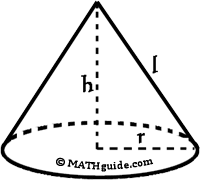General Cone

Example 1: Given r = 4 m and h = 3 m.

First we must calculate the slant height using The Pythagorean Theorem.
(4 m)2 + (3 m)2 = l2
16 m2 + 9 m2 = l2
25 m2 = l2
l = 5 m

The surface area would then require use of the formula...
SA = πr2 + πrl
SA = (π)(4 m)2 + (π)(4 m)(5 m)
SA = (16 m2)(π) + (20 m2)(π)
SA = 36π m2 (in terms of π) or
SA = 36(3.14159) m2
SA = 113.09724 m2
SA = 113.1 m2 (rounded to the nearest tenth)

Example 2: Given r = 7 in and h = 10 in.

First we must calculate the slant height using The Pythagorean Theorem
(7 in)2 + (10 in)2 = l2
49 in2 + 100 in2 = l2
149 in2 = l2
l = 12.21 in

The surface area would then require use of the formula...
SA = πr2 + πrl
SA = (π)(7 in)2 + (π)(7 in)(12.21 in)
SA = (49 in2)(π) + (85.47 in2)(π)
SA = 134.47(π) in2
SA = 134.47(3.14159) in2
SA = 422.44961 in2
SA = 422.4 in2 (rounded to the nearest tenth)ideo: Surface Area of a Coneuizmaster: Surface Area of Conesctivity: Cone Suface Area

SA = 4πr2General Sphere

Example 1: Given r = 3 ft.

The surface area requires the use of the formula...
SA = 4πr2
SA = 4(π)(3 ft)2
SA = 4(π)(9 ft2)
SA = 36π ft2 (in terms of π) or
SA = 36(3.14159) ft2
SA = 113.09724 ft2
SA = 113.1 ft2 (rounded to the nearest tenth)

Example 2: Given diameter = 20 m or r = 10 m.

The surface area requires the use of the formula...
SA = 4πr2
SA = 4(π)(10 m)2
SA = 4(π)(100 m2)
SA = 400π m2 (in terms of π) or
SA = 400(3.14159) m2
SA = 1256.636 m2
SA = 1256.6 m2 (rounded to the nearest tenth)ideo: Surface Area of a Sphereuizmaster: Surface Area of Spheres

 Instructional Videos Use these videos to solidify your understanding of these solids.ideo: Surface Area: Rectangular Prismsideo: Surface Area: Cylindersideo: Surface Area: Square-Based Pyramidsideo: Surface Area of a Coneideo: Surface Area of a Sphere Quizmasters After reading the lessons, try our quizmasters. MATHguide has developed numerous testing and checking programs to solidify these skills:uiz: Surface Area of Prismsuiz: Surface Area of Cylindersuiz: Surface Area of Pyramidsuiz: Surface Area of Conesuiz: Surface Area of Spheres Activities Here are activities related to the lessons above.ctivity: Cone Suface Areactivity: Cylinder Surface Areactivity: Pyramid Surface Area Related Lessons Here are related lessons.esson: Areas of Common Figuresesson: Areas and Sides of Similar Figures